# Decision Making 1: Expected Value Theory

Before you start today's material, please complete this survey.

## Decision making

In this section of the class we will focus on making models of simple economic decisions - basically decisions about gambles of the kind you just made in the survey. (Seriously, if you didn't complete the survey please do so now - we'll be using your data for the rest of the class and it only takes a few minutes to complete - so take the survey!).
An example of a decision like this is whether to gamble on roulette.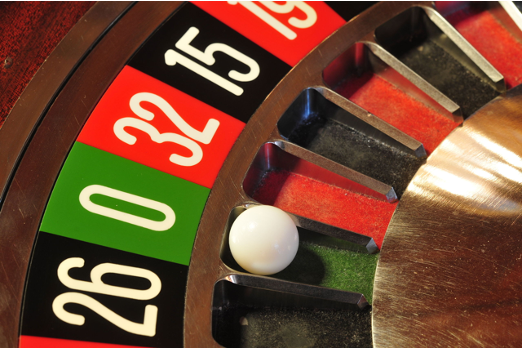For example, suppose you are considering betting $10 on red. If you win you double your money, if you lose you lose your money. What should you do? Well it very much depends how likely it is that the ball lands on red. If you are dealing with a standard European roulette wheel you will have 18 red numbers, 18 black numbers and 1 green number. So the probability of getting red is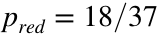and the probability of getting not-red is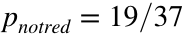So your decision is between these two options: • Don't play and get to keep$10 for sure
• Play and get $20 with probability 18/37 and get$0 with probability 19/37.
To model this decision we are going to focus on a very simple theory that says what you should do if you want to maximize your payoff on average. This theory is called Expected Value Theory.
Note: If you take my other classes (Judgment and Decision Making PSY 333 or Neuroeconomics PSY 433) you will see that Expected Value Theory is a really awful theory of human behavior and leads to all kinds of madcap predictions about behavior. However, for our purposes of building simple models in Matlab it does well enough. One possibility for a project for this class, however, would be to extend what we do in this section of the class to some more sophisticated theories like Expected Utility Theory and Prospect Theory.
Expected Value Theory says that you should base your decision on the average (or "expected") outcome associated with each gamble. Applied to our roulette problem this is pretty easy to compute.
The first option is not to play, in which case we keep $10 for sure. So the Expected Value of not playing is 10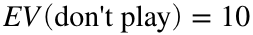The second option is a bit more complicated, but not much. What's the average outcome when we have a 18/37 chance of winning$20 and a 19/37 chance of winning $0? You might already have a feel for how to compute this, it's just the probability of winning multiplied by the amount you get if you win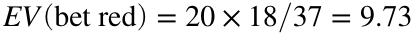Now, 9.73 is less than 10 so EV theory says you should not gamble in this case. As a corollary to this - the very existence of Las Vegas disproves that people use Expected Value Theory! More generally, we can imagine a more general gamble that has a bunch of different outcomes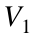,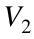, ...,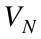each with it's own probability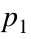,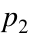, ...,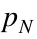. For example, this is the case if you play PowerBall - the jackpot is just one outcome (with very low probability), you can also get other outcomes for matching fewer balls all with different probabilities. In this general case the Expected Value is given by this equationi.e. we take the sum over all outcomes of the probability of each outcome times the value of that outcome. ## Compute Expected Values in Matlab There are lots of ways to compute Expected Values in Matlab, that is there are lots of ways to implement the EV equation above, but one particularly straightforward approach is to use vectors for the probabilities and the values. For example, in the roulette example we make a vector for probabilities like this p = [18/37 19/37]; and a vector for outcomes like this V = [20 0]; We can then compute the multiplication of these two terms like this for i = 1:length(p) pV(i) = p(i) * V(i); end and then finally we can compute the sum like this ... EV = sum(pV) EV = 9.7297 ### Element-wise multiplication This works well, but there's actually a way to skip the for loop part by performing element-wise multiplication of p and V. In particular, to compute the ith element of pV we multiplied the ith element of p by the ith element of V. We can actually do that in a single step like this ... pV = p.*V; This gives us exactly the same thing as the for loop but takes fewer lines and is actually faster for Matlab to run (not that you'll notice the difference in this case!). If you go down this route, be sure not to forget the . in the .* operator. The dot tells Matlab that you want to do element-wise multiplication. If you omit that you'll end up with matrix multiplication which is very different beast indeed ... ### Matrix multiplication If you omit the . in the .* and write p*V you will get an error. The reason is that Matlab is trying to implement a matrix multiplication. Specifically it's treating p as a 1 x 2 matrix (or a row vector) and V as a 1 x 2 matrix and trying to do this ...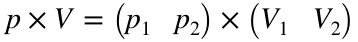Unfortunately this operation isn't permitted because the number of rows of p (i.e. 1) don't match the number of columns of V (i.e. 2) so Matlab throws an error. However, there is a way to make use of matrix multiplication in this example and go for the Expected Value directly. In particular we can compute the matrix multiplication of p and the transpose of V ...Taking the transpose of a matrix just switches the rows and columns. For a row vector it turns it into a column vector. This multiplication is allow and can be written asWhere the last term is the expression for the Expected Value! So, there's actually a way to do the multiplication and the sum to compute the expected value in just one line ... EV = p * V' EV = 9.7297 where the ' symbol tells Matlab to take the transpose of V. Be careful with matrix multiplication though, because if you take the transpose of the wrong thing you can get a very different answer. For example, if we take the transpose of p instead of V then we get something quite different ... EV = p' * V EV = 2×2 9.7297 0 10.2703 0 This is becauseOften in practice it's simpler to use the for loop and only move to elementwise or matrix multiplication if you need to speed things up! ## Expected Value Theory applied to the decisions in the survey OK, last chance! If you've not done the survey by now please do so - it only takes a few minutes. There are serious spoilers ahead so if you've not done it (and want to do it) this is your last chance! Let's apply Expected Value Theory to some of the decisions in the survey. In the Assignment, you will apply it to all of them. All of the questions were of this form, with one safe option and one risky option ... • Win X for sure (safe option) • Y% chance of$Z (risky option)
So the Expected Value of the safe option is always X = $10 X = 10; EV_safe = X; In one question, X was 25% and Y was$19.74. In this case, the Expected Value of the risky option is
Y = 0.25;
Z = 19.74;
EV_risky = Y * Z
EV_risky = 4.9350
This is less than $10 for sure so Expected Value Theory says we should always take the safe option. In Matlab we can create a variable "choice" which is 1 if the model chooses the safe option and 0 if it chooses the risky option ... choice = EV_safe > EV_risky choice = logical 1 So now we've got code to predict what Expected Value theory would do for any decision. Let's turn this into a function function [EV_safe, EV_risky, choice] = EVtheory_survey(X, Y, Z) EV_safe = X; EV_risky = Y * Z; choice = EV_safe > EV_risky; We can use the following line to compute the choice and EVs for any question on the survey. Let's do it for two of them, the one we've already done and another question where the risky option had a 75% chance of yielding$19.97 (in this case EV_risky will be higher than EV_safe)
[EV_safe(1), EV_risky(1), choice(1)] = EVtheory_survey(10, 0.25, 19.74);
[EV_safe(2), EV_risky(2), choice(2)] = EVtheory_survey(10, 0.75, 19.97);
Note: when you do something similar in the Assignment you may want to use a for loop ...
Now let's plot these choices on a "choice curve." Specifically, let's plot the difference in expected value between the safe and risky options on the x-axis and the choice (or rather the probability of choosing the safe option) on the y-axis ...
clf;
plot(EV_safe - EV_risky, choice, '.', 'markersize', 50)
xlabel('EV_safe - EV_risky', 'interpreter', 'none')
ylabel('probability of choosing safe')
set(gca, 'fontsize', 18)
xlim([-20 20])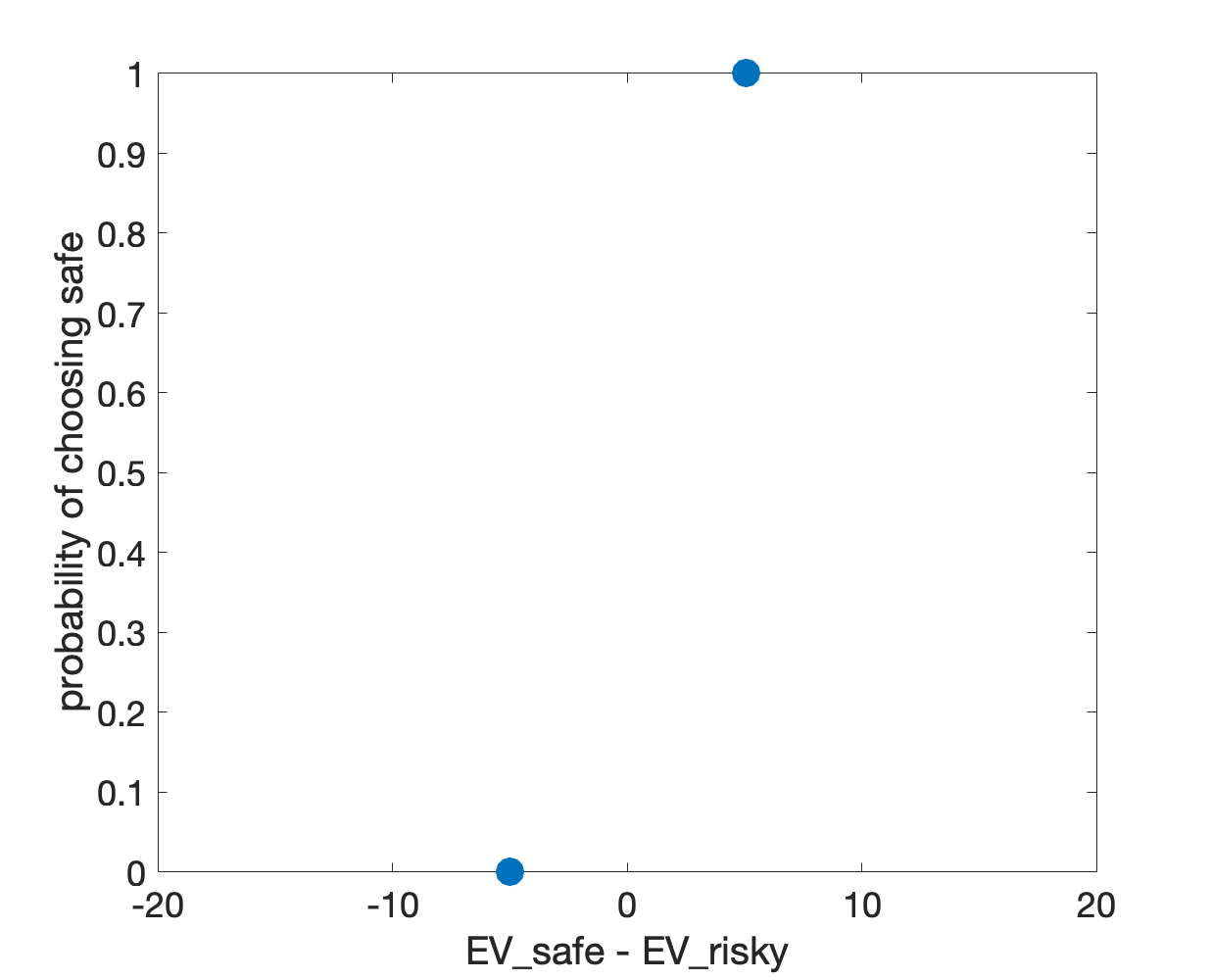Here you can see that the theory switches its preference from the safe option for the first question to the risky option for the second question.
More generally, the theory will always choose the safe option if EV_safe > EV_risky, and always choose the risky option if EV_risky > EV_safe. In this case the theoretical choice curve will look like a threshold function
clf;
plot([-20 0 0 20], [0 0 1 1], 'linewidth', 3)
xlabel('EV_safe - EV_risky', 'interpreter', 'none')
ylabel('probability of choosing safe')
set(gca, 'fontsize', 18)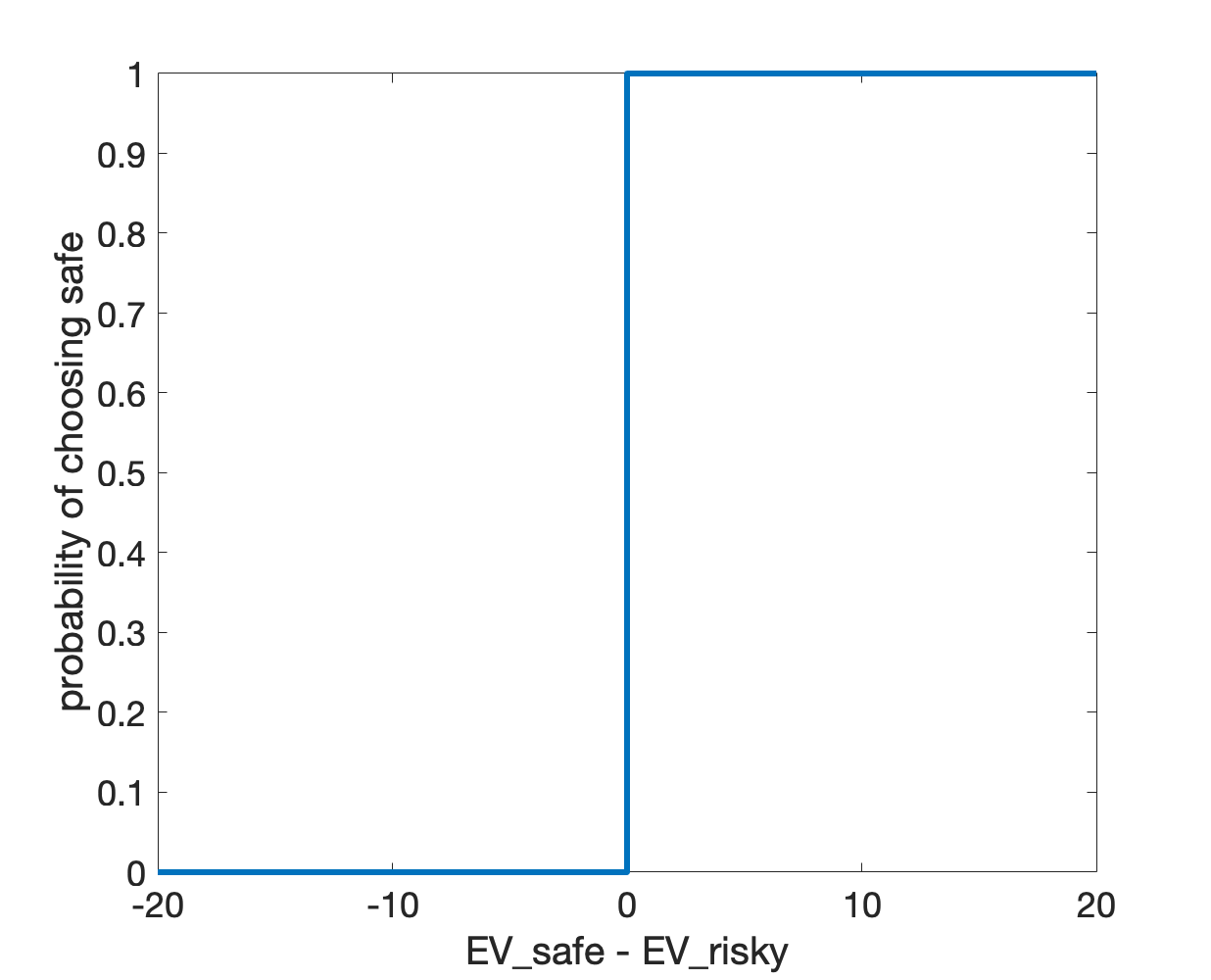However, this deterministic nature of pure Expected Value Theory is a problem. If you think about it, it gets pretty absurd pretty quickly ... imagine these two gambles, both in comparison to $10 for sure ... •$20 with 49% probability
• \$20 with 51% probability
In the first case, EV theory always picks the safe option. In the second case, EV theory always picks the risky option. Do we really believe that this will be the case for humans? It's unlikely and you'll see next week when you process data from the survey (sorry it's too late to do it now if you haven't done it!) that it's not at all what people do. Instead you'd expect people to choose the safe option with about the same probability for both options - and that probability should be somewhere between 0 and 1. So how can we implement this with a model? The answer is by adding noise ...

## Expected Value Theory with noise

If I just base my decisions on pure Expected Values, my behavior will be completely deterministic and I will switch from always choosing one option to always choosing the other based on just tiny changes in probabilities or values. Instead, we'd like a theory that is a bit more fuzzy. Yes it should prefer options with higher expected values, but it shouldn't always choose the high expected value option, especially when the expected values of the two options are similar.
One way to get this behavior is to add random noise to the expected values. The idea here is that random noise can, by chance, get us to choose a low Expected Value option with some probability. So instead of basing our decision on the pure expected value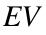, we base it on the noisy expected value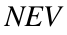In our case we will assume that the noise is Gaussian with a standard deviation σ, which for now we will set to 5
sigma = 10;
To get Gaussian noise we use the "randn" function. randn gives a Gaussian random number with mean 0 and standard deviation of 1. If we multiply it by sigma, we get a Gaussian random number with a mean of zero and a standard deviation of sigma ...
noise = randn(1) * sigma;
Note: the (1) when we call randn tells Matlab to give us 1 Gaussian random number.
We then just need to add noise like this to both of our expected values.
NEV_risky = EV_risky + randn(1) * sigma;
NEV_safe = EV_safe + randn(1) * sigma;
Note: This code will add different noise values to EV_safe and EV_risky.
Then, we compute the choice based on the noisy EVs ...
choice = NEV_safe > NEV_risky;
Finally, let's stick all this code into a function to make it easier to use
function [EV_safe, EV_risky, choice, NEV_safe, NEV_risky] = noisyExpectedValueTheory(X, Y, Z, sigma)
% compute noiseless expected values
EV_safe = X;
EV_risky = Y * Z;
% compute noisy expected values
NEV_risky = EV_risky + randn(1) * sigma;
NEV_safe = EV_safe + randn(1) * sigma;
% compute choice
choice = NEV_safe > NEV_risky;

## The behavior of Noisy Expected Value Theory

Let's compute the average behavior of the Noisy Expected Value theory model. Note that to compute the average behavior we will need to simulate a bunch of choices for the each gamble ...
clear
sigma = 10;
for i = 1:100
[EV_safe, EV_risky, choice(i)] = noisyExpectedValueTheory(10, 0.25, 19.74, sigma);
end
p_safe = mean(choice)
p_safe = 0.5900
Great! Our choice probability is less than 100%. Let's put this for loop inside a function just to make things easier when we compute p_safe for a bunch of different questions
function [EV_safe, EV_risky, p_safe, choice] = NEVtheory(N, X, Y, Z, sigma)
for i = 1:N
[EV_safe, EV_risky, choice(i)] = noisyExpectedValueTheory(X, Y, Z, sigma);
end
p_safe = mean(choice);
where N let's us input how many decisions we want to simulate.
Note I'm also outputting the noiseLESS expected values - these are the experimental values an experimenter knows about (the noise is presumably in the subject's head) and what we will put on the x-axis in the choice-curve plot.
Note: In addition I'm outputing the simulated choices. We won't use this output until Week 10, but when we do it will be super useful to have it so I'm putting it in here now ...
N = 100;
sigma = 5;
[EV_safe(1), EV_risky(1), p_safe(1)] = NEVtheory(N, 10, 0.25, 19.74, sigma);
[EV_safe(2), EV_risky(2), p_safe(2)] = NEVtheory(N, 10, 0.75, 19.97, sigma);
Note: again when you come to do this in the Assignment for all the survey questions, you may want to use a for loop to speed things up ...
Then we can plot the two points on the choice curve like this ...
clf;
plot(EV_safe - EV_risky, p_safe, '.', 'markersize', 30)
xlabel('EV_safe - EV_risky', 'interpreter', 'none')
ylabel('probability of choosing safe')
set(gca, 'fontsize', 18)
xlim([-20 20])
ylim([0 1])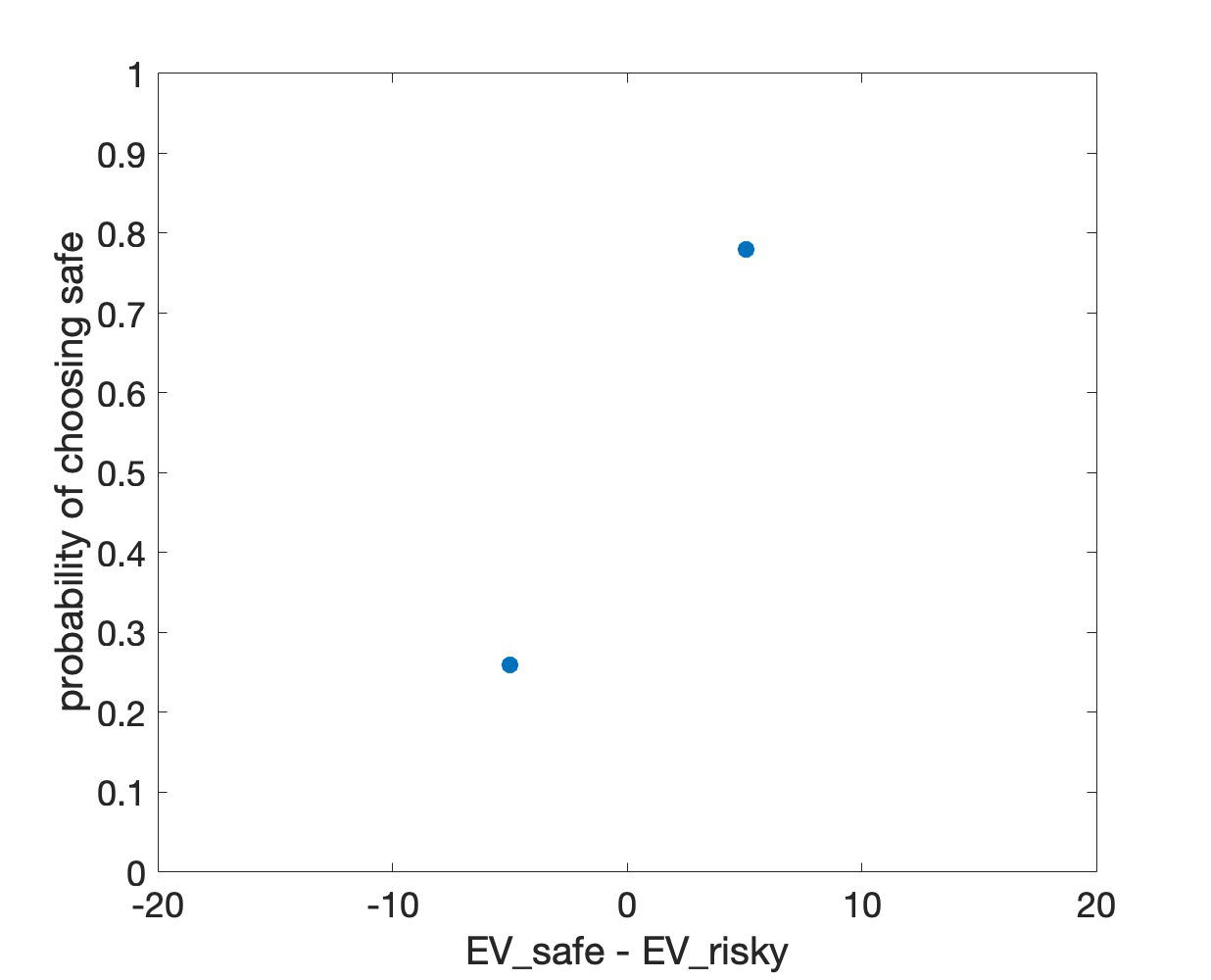Note that the probabilities are now in between 0 and 1. You will see in the Assignment, when you map out more of the curve, that as the difference between EV_safe and EV_risky gets smaller, the choice probabilities get closer to 50% and the resulting choice curve varies smoothly between (close to) 0 on the far left to (close to) 1 on the far right.

## The softmax function

It turns out that for the above model we can actually derive an analytic form (i.e. an equation) for the choice probability as a function of the Expected Values. In particular, we can write the probability of choosing the safe option asThis is called the softmax choice function and is a very popular model of choices.
Note: the softmax function is actually an approximation to the choice curve for Gaussian noise, but it's a pretty good one.
The softmax choice function has one free parameter, β. This parameter is something we can vary to fit an individual's (or a group's) behavior and it is related to the standard deviation of the noise, σ, asIn the Assignment you will play around with different values of β to demonstrate how it changes the choice curve and (hopefully) get an appreciation for why it's called the softmax function.
Here we will evaluate the curve and plot it for σ = 10 and compare it to the two points we evaluated by simulation.
First let's compute β from σ
beta = 1 / sigma * sqrt(pi / 2);
Now let's define a variable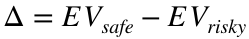as the different values of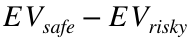we want to consider on the x-axis
Delta = [-20:0.1:20];
Finally, substitute it into the softmax expression for each value of Delta...
for i = 1:length(Delta)
p_safe_softmax(i) = 1 / ( 1 + exp( -beta * Delta(i)));
end
Note the minus sign before the beta, this is important so we compute p_safe
If we are feeling brave, we can replace the for loop using elementwise division ...
p_safe_softmax = 1 ./ ( 1 + exp( -beta * Delta ) );
Finally, let's stick it all in a function to make our final script cleaner
function p_safe_softmax = softmax(sigma, Delta)
% compute beta
beta = 1 / sigma * sqrt(pi / 2);
% compute softmax choice probabilities
p_safe_softmax = 1 ./ ( 1 + exp( -beta * Delta ) );
So we can call this from our script
p_safe_softmax = softmax(sigma, [-20:0.1:20]);
and plot it like this ...
clf;
plot(Delta, p_safe_softmax, 'linewidth', 3)
hold on
plot(EV_safe - EV_risky, p_safe, '.', 'markersize', 30)
xlabel('EV_safe - EV_risky', 'interpreter', 'none')
ylabel('probability of choosing safe')
set(gca, 'fontsize', 18)
xlim([-20 20])
ylim([0 1])